Next: 5 Experimental Details Up: 4.4 Field Distribution of Previous: 4.4.1 Vortex Lattice in

## 4.4.2 Vortex Lattice in a d-Wave Superconductor

The problem of an isolated vortex line in a dx2-y2-wave superconductor was first seriously considered by Soininen et al. , using a simple microscopic model for electrons on a lattice in the BdG formalism. In calculating the spatial distribution of the order parameter for a single vortex, they found that an s-wave component is induced near the vortex core with opposite winding of phase relative to the d-wave component. Several authors [167,168,169,170,171] have studied the effect of this induced s-wave order parameter on both an isolated vortex and the vortex-lattice structure, in terms of two-component GL equations containing both s-wave and d-wave order parameters. In these equations the s-wave component couples to the d-wave component through mixed gradient terms. Because of this coupling, the s-wave component is induced by spatial variations in the d-wave order parameter which occur in the vicinity of a vortex line. In a tetragonal superconductor the induced s-wave order parameter has fourfold symmetry and the d-wave order parameter has circular symmetry. Thus, in the core region of an isolated vortex, the magnetic field distribution is fourfold symmetric, whereas away from the core region, where the s-wave component vanishes, the field distribution has circular symmetry. At low temperatures near Hc2, the vortex lattice is oblique--reflecting the fourfold symmetry of the s-wave order parameter. However, near Tc the s-wave component becomes negligible and the vortex lattice is triangular. This latter prediction is crucial to the study of YBa2Cu3O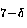in this thesis. Later it will be shown that when YBa2Cu3O6.95 is cooled through Tc in the presence of a magnetic field, the vortex lattice becomes strongly pinned at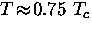and remains so for further reductions in temperature. Thus the vortex lattice geometry at low temperatures is governed by the geometry at the pinning temperature--which is nearly triangular in the two-component GL model.

It is well known that the orthorhombic crystal structure of YBa2Cu3Oresults in a significant mass anisotropy in the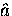-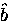plane--although the actual value of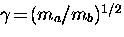is clearly a doping-dependent quantity. For instance, according to infrared reflectance measurements at zero field: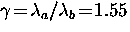and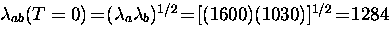Å  in YBa2Cu3O6.95 , while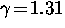and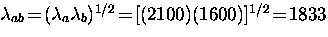Å  in YBa2Cu3O6.60 single crystals  similar to those used in the present study. Xu et al.  have extended the two-component GL theory to include the effects of mass anisotropy. When the magnetic field is applied parallel to the crystallographic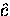-axis, both the s-wave and d-wave order parameters show a two-fold symmetry, where the d-wave order parameter has essentially elliptical symmetry. Within the GL formalism, Heeb et al.  find a similar reduction from fourfold to twofold symmetry in the presence of orthorhombic distortions. More recently, Ichioka et al.  reconstructed the two-component GL theory to investigate the vortex lattice in a pure dx2-y2-wave superconductor at low temperatures near Hc2. These authors argue that correction terms derived from the Gor'kov equations which are absent in conventional GL theory must be included at low T. They find that the unit-cell shape of the vortex lattice transforms from hexagonal to square at low temperatures, with the fourfold symmetry of the cores becoming clearer, even when there is no induced s-wave component included in the theory.

It is important to realize that the results using the two-component GL theory are strictly valid only along the superconducting-to-normal phase boundary near Hc2, and therefore do not necessarily provide an understanding of the vortex-lattice structure deep in the superconducting state where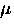SR experiments are performed. Near Hc2, where the vortices are close together, the fourfold symmetry of the induced s-wave component in the cores leads to a fourfold symmetry in the vortex-lattice configuration. However, there is no reason to expect this to be the case at low fields where the density of vortices in the superconductor (and hence the influence of the cores on the flux-lattice geometry) is diminished. For instance, in the borocarbide superconductors RNi2Bi2C (R= Er, Lu) the vortex lattice has been shown to transform from square to triangular at low fields [176,177]. Although the origin of the fourfold symmetry at high fields is as yet unresolved in this family of compounds, it is clear that the geometry of the vortex lattice can change with reduced vortex-vortex interactions. Of course all of this is irrelevant if the vortex lattice freezes'' in at high temperatures, as mentioned earlier.

Another serious problem with the two-component GL theory, is that it contains too many phenomenological parameters to be useful in fitting the measured internal field distributions. Recently, Affleck et al.  attempted to resolve these issues by generalizing the London model to include four-fold anisotropies in a tetragonal material. Starting from a GL free energy density with s and d-wave order parameters, they derived the corresponding London equation. For a magnetic field applied along the-axis, the field profile which is obtained may be written as 

where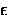is a dimensionless parameter which controls the strength of the coupling between the s and d-wave components. For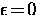, Eq. (4.17) reduces to Eq. (4.10). Unfortunately, the vortex-lattice structure obtained by minimizing the Gibbs free energy of Eq. (4.15) depends on the choice of,which cannot be determined independently.

Franz et al.  have recently developed a generalized London model derived from a simple microscopic model, which takes into account the nonlocal behaviour which occurs in the vicinity of the nodes in a dx2-y2-wave superconductor. This modified London model predicts novel changes in the vortex-lattice geometry, including two first order phase transitions at low T. More recently, this work has been extended to account for both nonlinear and nonlocal effects as discussed earlier . It is found that the nonlocal corrections are the dominant effect in determining the vortex-lattice geometry. In particular, the numerical calculations in Ref.  yield a nearly triangular vortex lattice. It should be noted, however, that the source term the authors used in the London equation was derived from the GL equations near Hc2 , and is not theoretically valid for lower magnetic fields.

Shiraishi et al.  have studied the vortex lattice using the extended GL theory, which includes the fourth-order derivative term and accounts for the finite size of the vortex cores. The fourfold symmetry of the vortex cores leads to a first order transition in the vortex-lattice geometry with increasing magnetic field. In particular, in weak fields the vortex lines form a triangular lattice which slowly transforms with increasing magnetic field, and then suddenly changes to a square lattice. Near Tc they predict a crossover field given by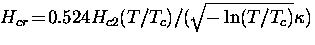.

The structure of a single isolated vortex in a pure dx2-y2-wave superconductor has been calculated using the quasiclassical Eilenberger theory [181,182], which is valid at arbitrary temperature. A fourfold symmetry appears in the LDOS, the pair potential, the supercurrent and the magnetic field distribution around a vortex. The fourfold symmetry about the vortex center is strongest in the core region and gradually fades to circular symmetry toward the outer region. On the other hand, using an approximate version of the BdG equations Morita et al.  found that the LDOS around a single dx2-y2-wave vortex has circular symmetry, and exhibits fourfold symmetry only when an s-wave component is mixed in. Franz and Ichioka  have since argued that the circular symmetry obtained by these authors is an unphysical artifact of the approximations used for the BdG equations. The BdG equations have been solved numerically for a vortex lattice of a d-wave superconductor [166,185]. Unfortunately, there are currently no calculations (in any formalism) of the vortex-lattice structure in a dx2-y2-wave superconductor, which are valid at both low T and low H where experiments are generally performed.

Several authors [182,185,186] have suggested that the low-lying quasiparticle excitations cannot be bound in a d-wave vortex core because of the presence of the nodes. Rather than states which are localized in the core as in a s-wave superconductor, the states are peaked in the core region but extend along the node directions. According to Ichioka et al. , in the vortex state of a dx2-y2-wave superconductor the quasiparticles do not flow along conventional closed circular trajectories, but rather flow along open trajectories which connect with those of nearest-neighbor vortices. This theoretical model requires that the nodes lie along the line connecting nearest-neighbor vortices. In the absence of anisotropy, this implies that the nearest-neighbor direction must be 45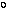.

So far, experiments have not entirely resolved the issue of the vortex-lattice structure in the high-Tc materials either. The major problem has been in determining how much of the observed vortex structure is directly attributable to the dx2-y2-wave pairing state and how much is due to deformations of the lattice caused by extrinsic effects. Generally speaking, Bitter decoration experiments which image the vortex lattice at the sample surface indicate that the vortices arrange themselves to form a triangular lattice. For instance, Gammel et al.  observed a triangular lattice with long-range order in YBa2Cu3O7. Decoration experiments by Dolan et al.  show a triangular lattice in YBa2Cu3O7, with a slight distortion probably caused by the-plane anisotropy. A triangular vortex lattice with long-range order was also observed by Vinnikov et al.  in Tl2Ba2CaCu2Ox at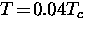and in Bi2Sr2CaCu2O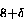by Kim et al.  at high temperatures. Since the Bitter decoration technique is resolution limited to low magnetic fields, the results obtained may not be representative of the lattice structure at higher magnetic fields, particularly in a sample dominated by extrinsic effects.

The structure of the vortex lattice in the bulk of a superconductor can be investigated using small-angle neutron scattering (SANS). The pattern generated by neutrons scattering from the vortex lattice is the reciprocal lattice of the real-space vortex lattice. Large single crystals are generally required so that the diffracted neutron intensity is strong enough to clearly resolve the peaks resulting from Bragg reflection. The scattered intensity is proportional to the square of the spatial variation in the local magnetic field, which is of the order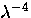. In principle, one can measure the temperature dependence of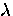using SANS. The signal-to-noise ratio is reduced in samples which contain defects which scatter the neutrons in the same small angle as those scattered from the vortex lattice. In clean conventional superconductors like Nb and NbSe2, a perfect triangular lattice with long-range order is observed using SANS [165,192]. A triangular lattice has also been observed in the high-Tc superconductor Bi2Sr2CaCu2Oat low temperatures . On the other hand, resolving the vortex-lattice geometry in YBa2Cu3Ohas been more difficult. Forgan et al.  investigated the vortex lattice in small single crystals of YBa2Cu3O7 up to fields of 0.6 T. Only diffraction spots corresponding to vortices parallel to the twin boundaries were strong. Relatively weak diffraction spots were observed in the other directions--which implies that the vortex lattice was non-uniform. A diffraction pattern with square symmetry was observed by Yethiraj et al.  in a SANS study of the vortex lattice in YBa2Cu3O7. The authors attribute the observed geometry to twin planes, since the intensity peaks are aligned along the (110) direction. More recently, Keimer et al.  studied the vortex lattice in a larger single crystal of YBa2Cu3O7 for magnetic fields of 0.5 to 1.5 T applied along the crystallographic-axis, at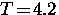K. These authors reported that the vortices form an oblique (fourfold symmetric) lattice with an angle of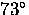between two nearly equal primitive vectors, and that one of the primitive vectors is oriented at an angle of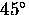with respect to either theoraxis. The alignment of one primitive vector of the oblique lattice with the (110) or (1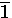0) direction of the underlying crystal lattice was observed in four different orientational domains of the crystal. Walker and Timusk  noted that the vortex-lattice geometry observed in this SANS experiment is easily explained as a combination of strong pinning effects due to twin planes and the-plane anisotropy in YBa2Cu3O7. In particular, an equilateral-triangular vortex lattice with one side aligned along a twin boundary, which is then stretched (due to the-plane anisotropy) along a line which makes aangle with the twin boundary, yields the observed vortex-lattice geometry.

An oblique lattice was also found in STM measurements performed by Maggio-Aprile et al.  on the (001) surface of twinned YBa2Cu3O, at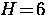T andK. Consistent with the SANS results, they report an angle of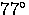between nearly equal primitive vectors--although they could not determine the orientation of the vortex lattice with respect to the crystal lattice. The oblique lattice imaged in this experiment was only observed locally, with no apparent long-range order. These authors also report that the vortex cores are ellipsoidal in shape with the ratio of the principle axes being about 1.5. The elongation of the cores is consistent with the-plane anisotropy in YBa2Cu3O--i.e. other than this anisotropy the vortex cores appear to be approximately circular. As is the case for the SANS experiments, the geometry of the observed vortex lattice can also be explained as a combination of the-plane anisotropy and an alignment of vortex lines with twin boundaries. Thus in a detwinned or sparsely twinned sample of YBa2Cu3O, it is likely that the vortex lattice is triangular at moderate magnetic fields.

There are several serious discrepancies between the current experiments on high-Tc superconductors in the vortex state and the theoretical models for the vortex lattice of a dx2-y2-wave superconductor:

1.
None of the current theoretical models can explain, in terms of an intrinsic mechanism, both the vortex-lattice geometry (observed in the SANS and STM experiments on YBa2Cu3O) and the orientation of the vortex lattice with respect to the crystallographic axis (observed in SANS experiments in YBa2Cu3O). To fully understand the influence that the symmetry of the pairing state has on the vortex-lattice geometry, it will be necessary to perform imaging experiments on untwinned crystals and/or tetragonal superconductors.
2.
The STM image of the vortex core on YBa2Cu3Oby Maggio-Aprile et al.  does not show the fourfold anisotropy predicted for the LDOS near a dx2-y2-wave vortex.

3.
Experiments performed on both YBa2Cu3O[197,198] and Nd1.85Ce0.15CuO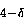are more consistent with a picture in which a few bound quasiparticle states exist in the vortex core--which contradicts the idea that the low-lying quasiparticle excitations cannot be bound in a dx2-y2-wave vortex core because of the nodes. For instance, in the STM experiment on YBa2Cu3Otwo peaks separated by about 11 meV were observed in the differential conductance dI/dV (i.e. the LDOS) measured at the center of a vortex core. A natural interpretation of this result is that these peaks correspond to the lowest bound quasiparticle energy levels.

Franz and Tesanovic  have recently attempted to address some of these issues by proposing a mixed dx2-y2+idxy pairing state. For this symmetry there is a finite energy gap everywhere at the Fermi surface so that bound quasiparticle states can exist. Within a BdG formalism these authors predict near spatially-isotropic bound quasiparticle states--however, the size of the dxy component required to reproduce the gap observed in the tunneling conductance at the center of the vortex core in Ref.  may be difficult to reconcile with other experiments. For instance, the linear-T dependence of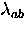found in our ownSR studies of YBa2Cu3Oin the vortex state [2,3,5] imply that any finite gap which opens along the node directions cannot be too large.

Given the anomalous normal state properties in the high-Tc compounds, it is quite reasonable to expect deviations from the conventional picture of a vortex core in these materials. One such novel description is the prediction from SO(5) theory for the existence of a superconducting vortex with an antiferromagnetic core in underdoped high-Tc compounds [200,201]. Recently, we have investigated this possibility in aSR study of YBa2Cu3O6.57 (Ref. ). However, neither this study or any other has produced clear evidence for the existence of antiferromagnetism in the vortex core.

With experiment and theory in apparent disagreement, it seems reasonable to model the vortex lattice in the simplest possible manner. Furthermore, it does not matter whether theory predicts a fourfold symmetric vortex lattice for YBa2Cu3Oif pinning freezes in'' the threefold (triangular) lattice just below Tc. In this thesis, the contribution of the vortex lattice to the measuredSR line shape will be modelled with both Brandt's modified London model [see Eq. (4.10)] and Yaouanc's version of Hao's analytic solution to the GL equations [see Eq. (4.13)]. The primary advantage of using these phenomenological models is that they contain a manageable number of parameters for fitting theSR spectra. In addition, both models are conveniently valid at low reduced fields b whereSR experiments are generally performed. I will show in this study that fitting to these models yields a good description of the T and H-dependences of the fundamental length scalesand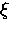(i.e. r0) in both conventional and unconventional superconductors.Next: 5 Experimental Details Up: 4.4 Field Distribution of Previous: 4.4.1 Vortex Lattice in